什么是决策树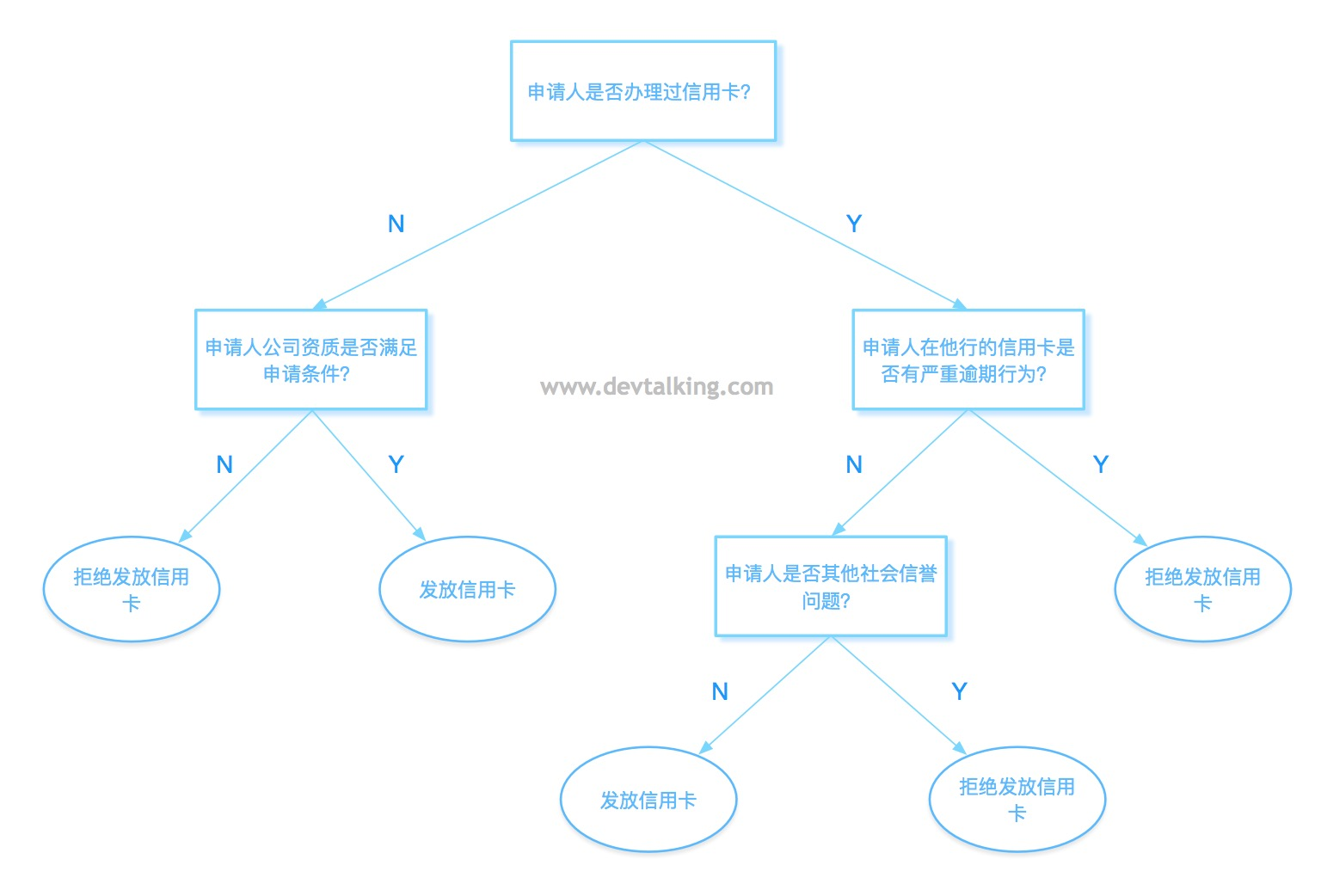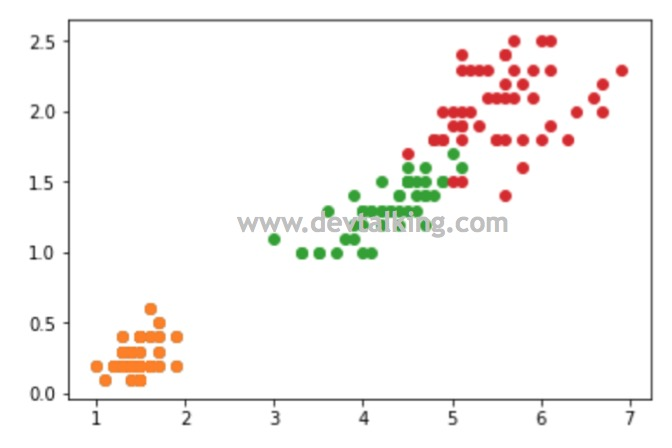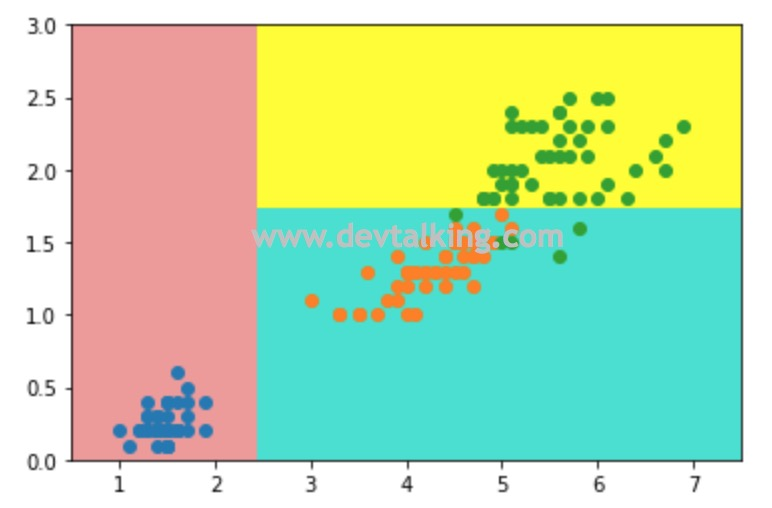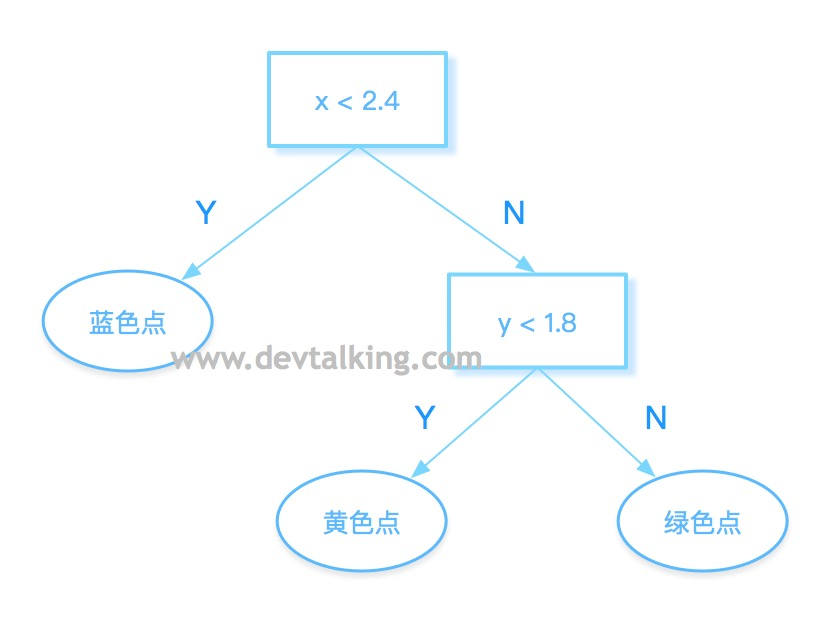• 决策树算法是非参数学习算法。
• 决策树算法可以解决分类问题。
• 决策树算法可以天然解决多分类问题。
• 决策树算法处理样本数据的结果具有非常好的可解释性。

信息熵

• 熵越大，数据的不确定性越高。
• 熵越小，数据的不确定性越低。

信息熵的公式

$$H = - \sum_{i=1}^k p_i log(p_i)$$

• 假如一组数据有k类信息，那么每一个信息所占的比例就是$p_i$。比如鸢尾花数据包含三种鸢尾花的数据，那么每种鸢尾花所占的比例就是$\frac 1 3$，那么$p_1$、$p_2$、$p_3$就分别为$\frac 1 3$。
• 因为$p_i$只可能是小于1的，所以$log(p_i)$始终是负数。所以需要在公式最前面加负号，让整个熵的值大于0。

$$\{\frac 1 3,\frac 1 3,\frac 1 3\}$$

$$H=-\frac 1 3 log(\frac 1 3)-\frac 1 3log(\frac 1 3)-\frac 1 3log(\frac 1 3)=1.0986$$

$$\{\frac 1 {10},\frac 2 {10},\frac 7 {10}\}$$

$$H=-\frac 1 {10} log(\frac 1 {10})-\frac 2 {10}log(\frac 2 {10})-\frac 7 {10}log(\frac 7 {10})=0.8018$$

信息熵曲线

$$H=-xlog(x)-(1-x)log(1-x)$$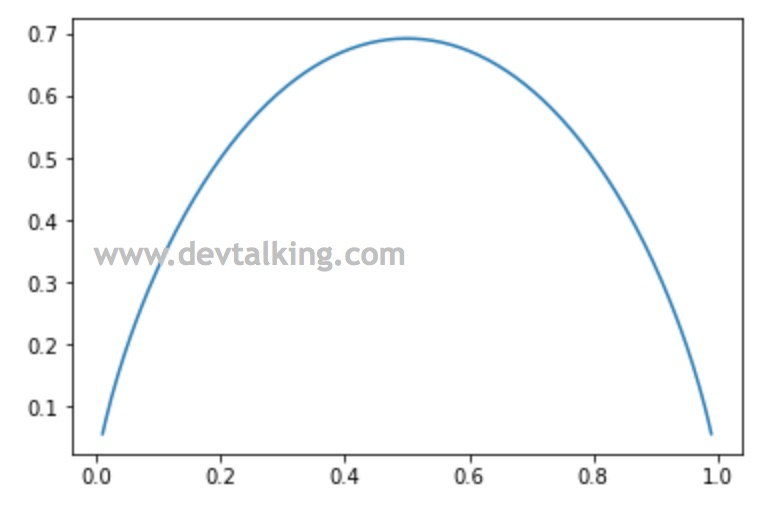使用信息熵寻找最优划分

• 决策树每个节点在哪个维度做划分？
• 某个维度在哪个值上做划分？

寻找维度和阈值函数